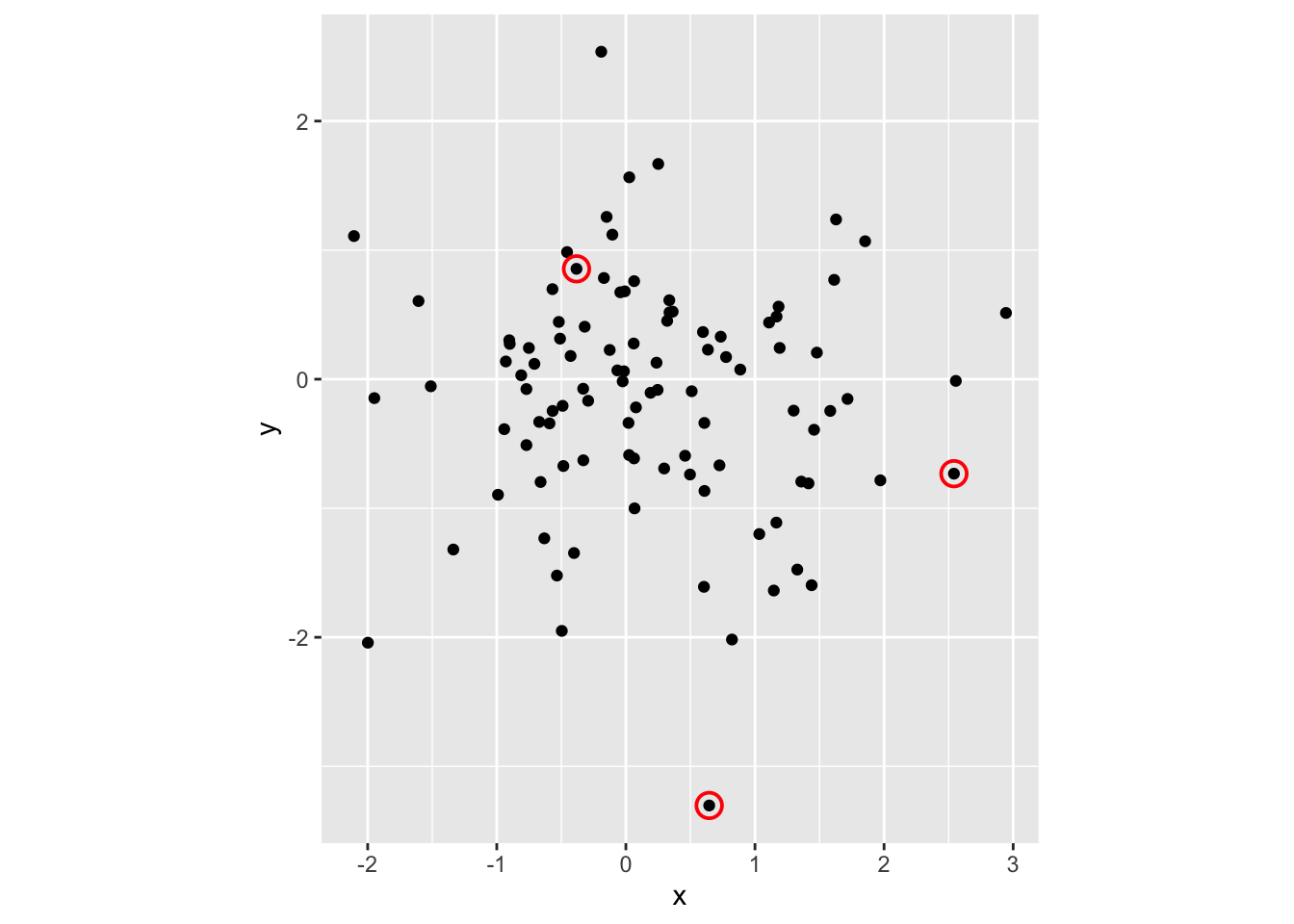# Highlight data points in a scatterplot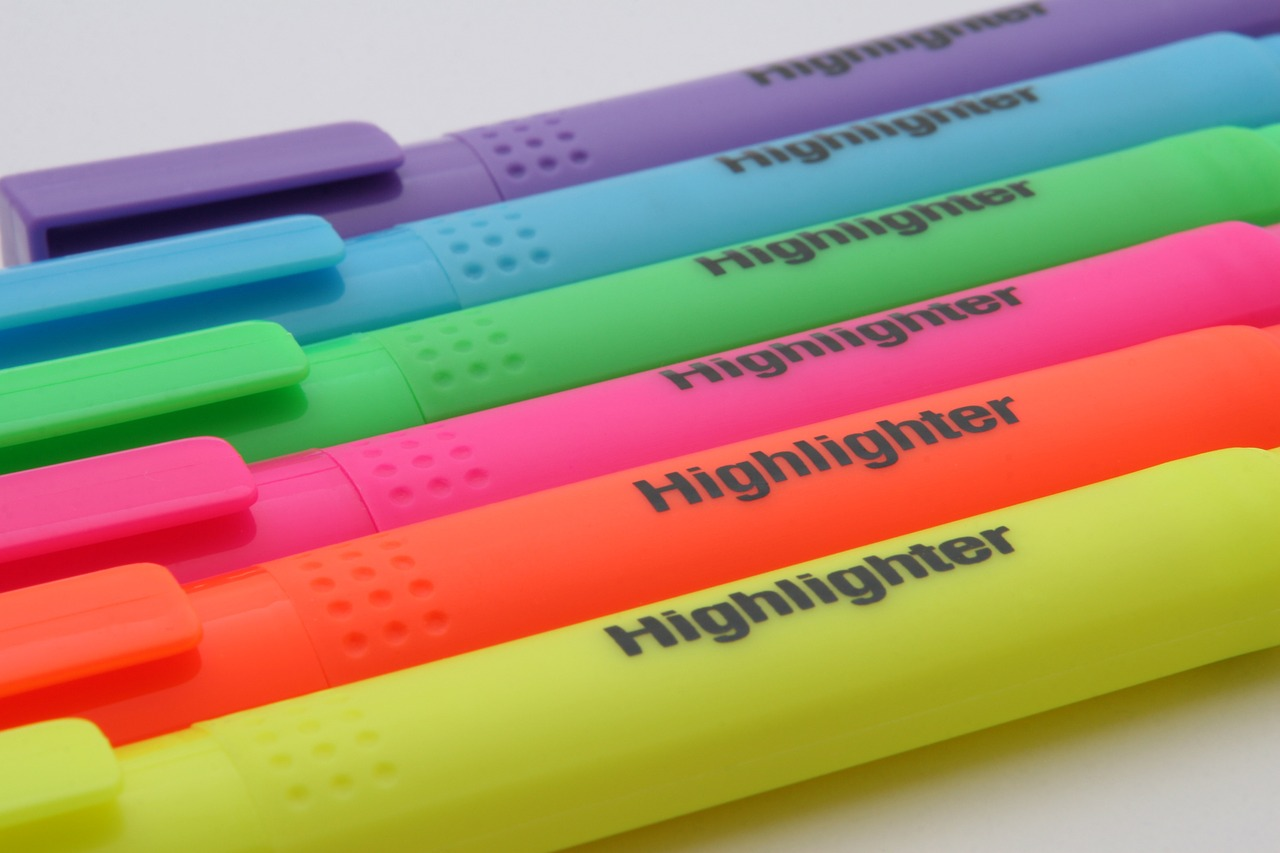library(tidyverse)

# Step 2: Dataset

set.seed(20201215)
df <- tibble::tibble(x=rnorm(100), y=rnorm(100))

Now we are going to work on our scatterplot.

# Step 3: First draw a scatterplot with your full data

ggplot(df, aes(x=x, y=y)) +
geom_point() +
coord_equal()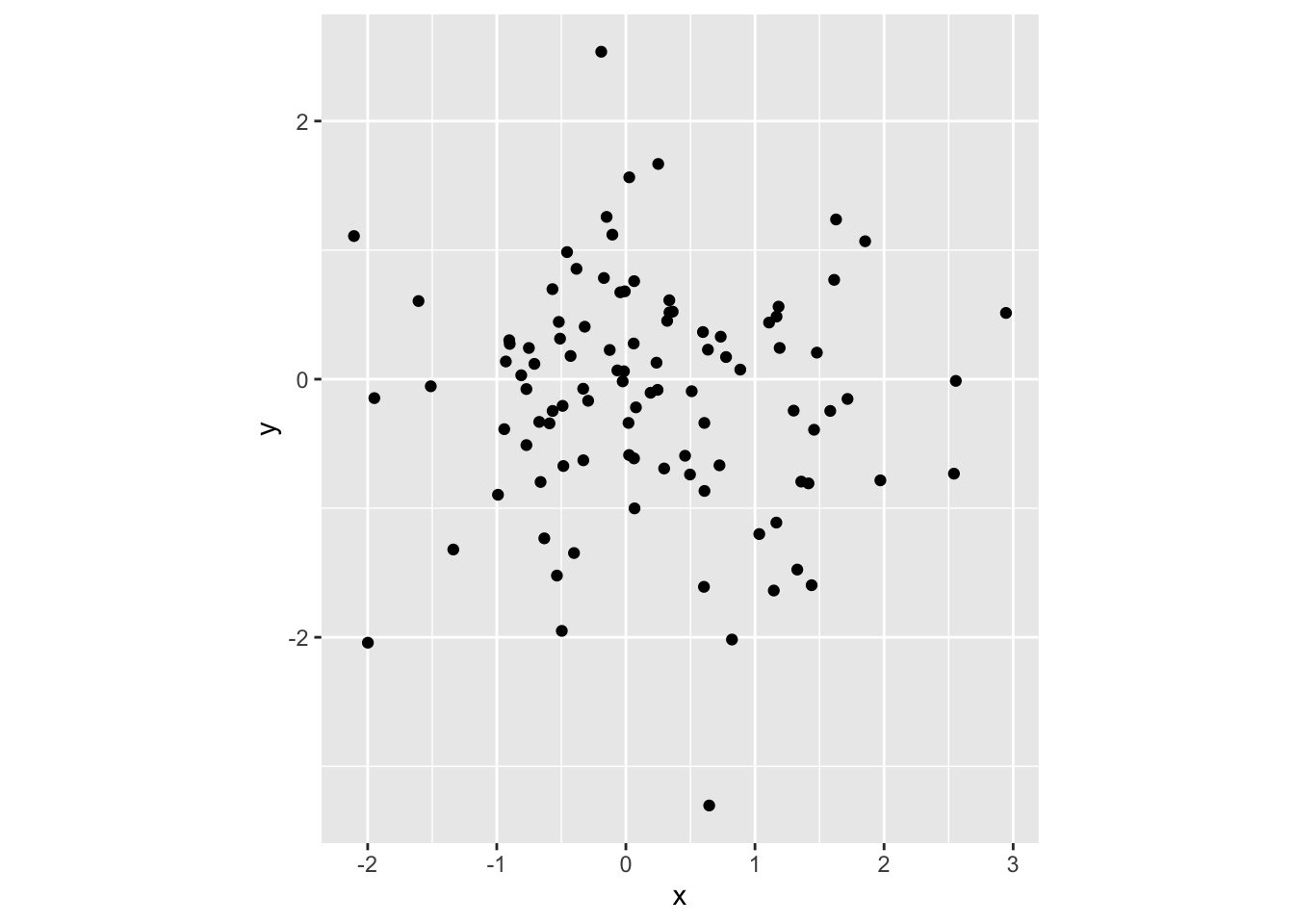# Step 4: Store the points that you need to highlight in a new data.frame or tibble.

Suppose I want to highlight 5th, 10th and 15th points.

df2 <- df[c(5, 10, 15), ]
df2
# A tibble: 3 x 2
x      y
<dbl>  <dbl>
1  0.646 -3.30
2  2.54  -0.732
3 -0.383  0.855

# Step 5: Now you need to inform above points to the ggplot through another geom_point layer.

ggplot(df, aes(x=x, y=y)) +
geom_point() +
coord_equal() +
geom_point(data=df2, aes(x=x, y=y), colour="red")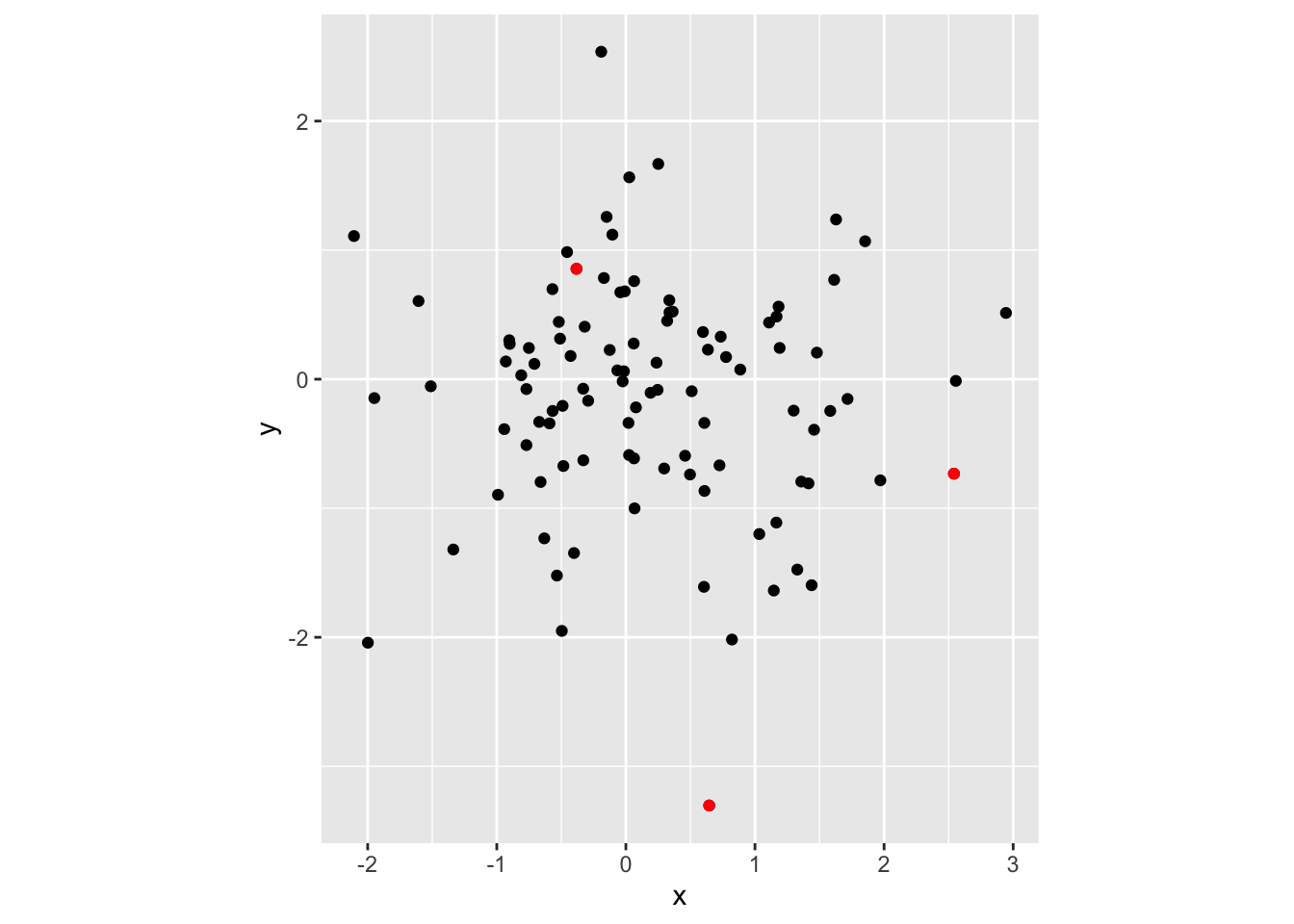We can change the size by passing a value to size argument.

ggplot(df, aes(x=x, y=y)) +
geom_point() +
coord_equal() +
geom_point(data=df2, aes(x=x, y=y),
colour="red",
size=5)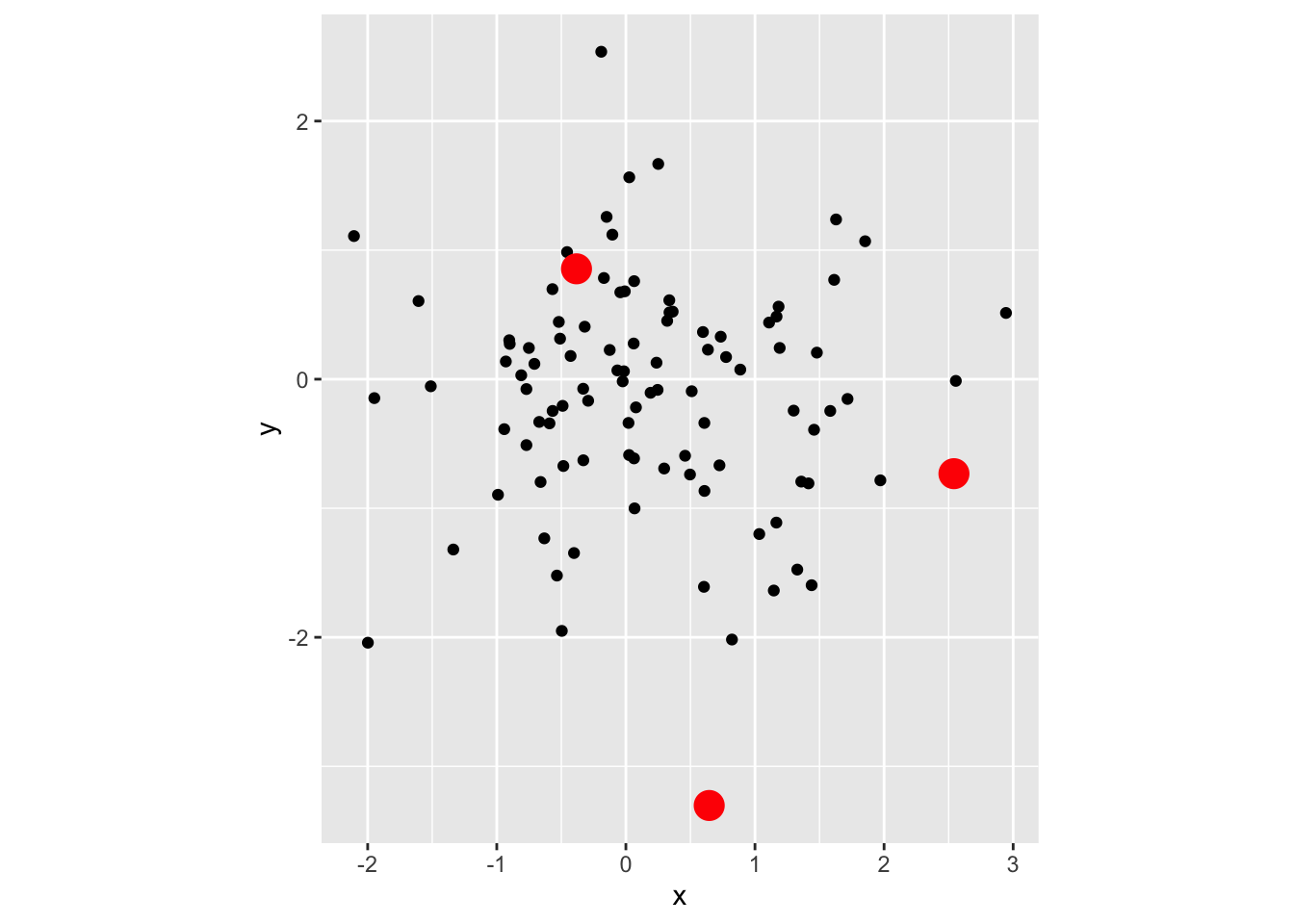We can circle around the points by using the following command.

ggplot(df, aes(x=x, y=y)) +
geom_point() +
coord_equal() +
geom_point(data=df2, aes(x=x, y=y), pch=21,
fill=NA, size=4,
colour="red", stroke=1)